Ch 7. Stress Analysis Multimedia Engineering Mechanics PlaneStress PrincipalStresses Mohr's Circlefor Stress Failure PressureVessels
 Chapter 1. Stress/Strain 2. Torsion 3. Beam Shr/Moment 4. Beam Stresses 5. Beam Deflections 6. Beam-Advanced 7. Stress Analysis 8. Strain Analysis 9. Columns Appendix Basic Math Units Basic Equations Sections Material Properties Structural Shapes Beam Equations Search eBooks Dynamics Fluids Math Mechanics Statics Thermodynamics Author(s): Kurt Gramoll ©Kurt GramollMECHANICS - THEORY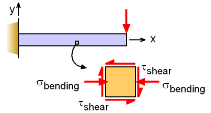Stresses at a point (Stress Element)
for a Cantilever Beam

In the previous sections, both the bending and shear stresses were presented for beams. Recall, the bending stress will cause a normal stress (either tension or compression, depending on vertical location) and the shear stress will cause a tearing stress. Both of the stresses can act at the same point and should be considered at the same time. Since only linear elastic materials are considered in this eBook, both stresses can be added together using the principle of superposition.

Stress Element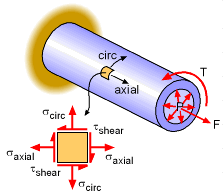Stresses at a point (Stress Element)
for Pressurized Pipe

The beam above is just one possible configuration for multiple stresses acting at a point. Another possibility is a pipe that is pulled, pressurized and twisted at the same time. These three loads on the pipe will cause tension normal stress in both directions (axial and circumferential) and cause a twisting or shear stress. All three loads and their associate stresses can be combined together to give a total stress state at any point.

The stress element for this example is shown at the left. This section will examine a stress element to better understand stresses at a point and how they can be analyzed.

Sign Convention for Stress Element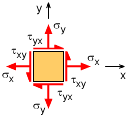Sign Convention for Stress Element
Positive Directions

The sign convention for stresses at a point is similar to other stresses. Normal tension stress in both the x and y direction are assumed positive. The shear stress is assumed positive as shown in the diagram at the left. Shear stress act on four sides of the stress element, causing a pinching or shear action. All shear stresses on all four sides are the same, thus

τxy = τyx

Stress Rotation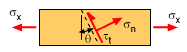Stress on an Inclined Plane

In the Normal Stress section, stress on an inclined plane was presented. It was noted that stresses are not vector quantities, and are not rotated by just using a single sin or cos function. For the normal and shear stress on an inclined plane, the rotated stresses were found to be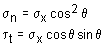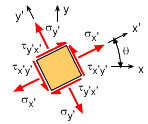Rotated Stress Element

Similarly, the stresses at a point (stress element) can also be rotated to give a new stress state at any particular angle. The rotation angle, θ, is assumed positive using the right hand rule (counter-clockwise in the x-y plane is positive). The new coordinate system is labeled as x' and y'. The new rotated stresses are shown in the diagram at the left. The shear stresses, τx'y' and τy'x, are still equal.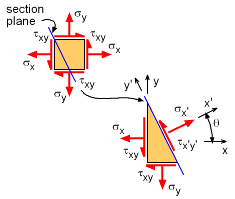Plane Section through Stress Element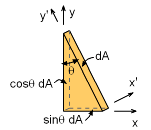Surface Area on Stress Element Plane

The objective is to relate the new stress in x' and y' coordinate system to the original stresses in the x and y coordinate. To do this, the original stress element is sliced at an angle θ, as shown in the diagram at the left. The stresses on the cut plane must be in equilibrium with the stresses on the outside surfaces of the stress element. Remember, nothing is moving, so all stresses and their associated forces must obey static equilibrium equations, ΣF = 0 and ΣM = 0.

Before the stresses are actually summed, the area on each surface needs to be defined. The plane section at the angle θ is assumed to have a basic area of dA. The stress element is really just a point, so the area is infinitesimal, or just dA. The other two surfaces are based on dA. The bottom surface will be 'sinθ dA' and the left surface will be 'cosθ dA', which are shown in the diagram at the left.

Summing the forces in each direction gives

ΣFx = 0 = (σ dA) cosθ - (τx´y´ dA) sinθ
- σx (cosθ dA) - τxy (sinθ dA)

ΣFy = 0 = (σ dA) sinθ - (τx´y´ dA) cosθ
- σy (sinθ dA) - τxy (cosθ dA)

There are two unknowns, σ and τx´y´ and two equations, so they can be determined, giving

σ = σx cos2θ + σy sin2θ + 2 τxy sinθ cosθ

τx´y´ = - (σx - σy ) sinθ cosθ +τxy (cos2θ - sin2θ)

The y' direction can be developed in the same way, but the section plane is 90o offset. The final equation is

σ = σx sin2θ + σy cos2θ - 2 τxy sinθ cosθ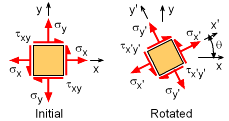Rotated Stress Element

Using double angle trigonometry identities, these three equations can be simplified to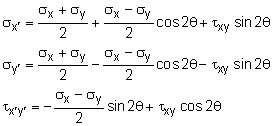Stress Rotation Plot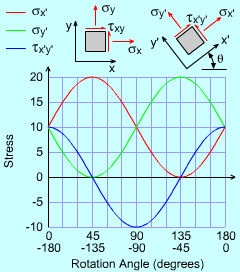Stress Rotation   To help visualize how the stress changes when the stress element is rotated, the diagram plots σ σ and τ   as a function of the angle. Notice, the period is 180o.

Practice Homework and Test problems now available in the 'Eng Mechanics' mobile app
Includes over 400 problems with complete detailed solutions.
Available now at the Google Play Store and Apple App Store.